# Notation for recurring decimals

Sometimes when you divide into a number there is a recurring decimal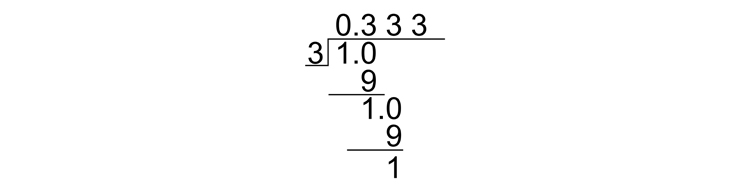1/3=0.333333.......recurring

This can be written as

1/3=0.dot3
or
1/3=0.bar3

The dot or dash means that the 3 is repeated indefinitely.

But repeated decimals can occur in a pattern.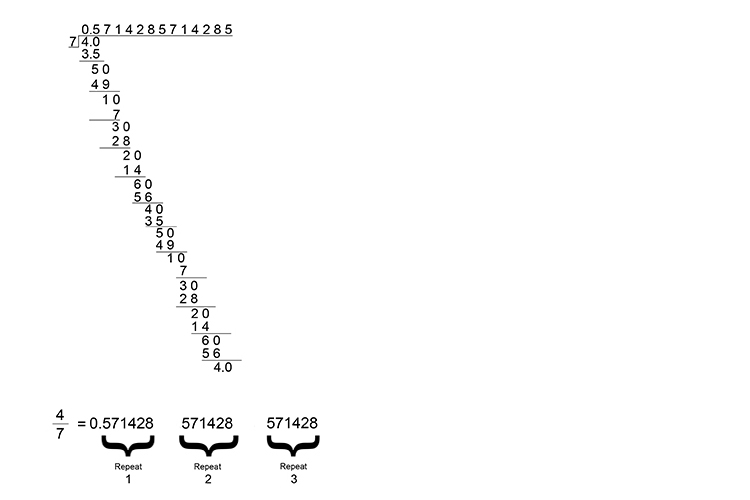This can be written as

4/7=0.dot5\714dot2   or  4/7=0.bar5\714bar2

This is written with a dot over the first and last part of the recurring pattern.

Example 1

Put the following fraction in recurring decimal form 22/7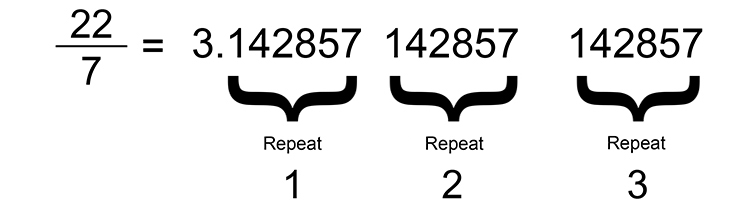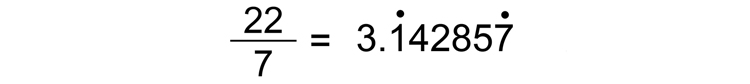Example 2

What does 1.dot5 mean?

1.dot5 means 1.5555555 repeating

Example 3

Write 22.520101010 as a recurring decimal using dots

This equals 22.520dot1dot0

Example 4

Express 4/9 as a decimal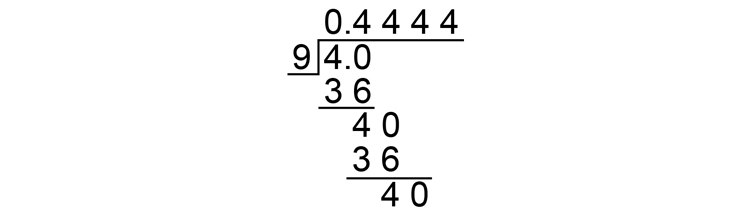4/9=0.4444 recurring

4/9=0.dot4